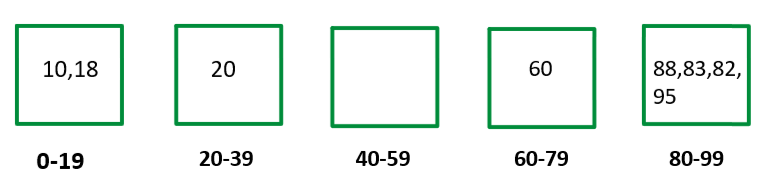# Bucket Sort : Detailed Explanation

### Introduction

You would wonder, why we need bucket sort even though we have sorting algorithms that work in O(nlogn). Bucket sort can be very useful when the data is uniformly distributed and has a certain range.

### What is bucket sort?

When a set of data is present within a range and uniformly distributed, we make buckets to store those elements. Later we sort those buckets and then join those sorted buckets. Now you would be wondering what these buckets are and how to visualize this.

Let’s try understanding it using a simple diagram

Suppose we have an array as follows:Here the elements range from 0-to 99

Now we create buckets. Suppose we choose 5 buckets, now we divide our range into 5 equal parts. We place the elements into the buckets according to the range in which the buckets are created.Now we sort these elements in the bucket individually.The final step, merging these buckets.This gives a rough idea of how bucket sort works. Now let’s try implementing bucket sort.

Implementation

For simplification, the number of buckets is pre-defined in the input.

Code:

## C++ Code

``````#include <bits/stdc++.h>

using namespace std;

int main() {
int arr[] = {30,40,10,80,5,12,70};

int k = 4;

int maxElement = arr;
int arrSize = sizeof(arr) / sizeof(arr);
for (int i = 1; i < arrSize; i++) {
maxElement = max(maxElement, arr[i]);
}
maxElement++;

// Building and filling buckets
vector < int > buckets[k];
for (int i = 0; i < arrSize; i++) {
int bucketIndex = (k * arr[i]) / maxElement;
buckets[bucketIndex].push_back(arr[i]);
}
// sorting buckets
for (int i = 0; i < k; i++) {
sort(buckets[i].begin(), buckets[i].end());
}
// merging buckets
int ind = 0;
for (int i = 0; i < k; i++) {
for (int j = 0; j < buckets[i].size(); j++) {
arr[ind++] = buckets[i][j];
}
}
for (int i = 0; i < arrSize; i++) cout << arr[i] << " ";
return 0;
}``````

output: 5 10 12 30 40 70 80

## Java Code

``````import java.io.*;
import java.lang.*;
import java.util.*;

class TUF {
public static void main(String[] args) {
int arr[] = {30, 40, 10, 80, 5, 12, 70};
int n = arr.length;
int k = 4;
bucketSort(arr, n, k);

for (int i = 0; i < n; i++)
System.out.print(arr[i] + " ");

}

static void bucketSort(int arr[], int n, int k) {

int maxElement = arr;
for (int i = 1; i < n; i++)
maxElement = Math.max(maxElement, arr[i]);
maxElement++;

// Building buckets
ArrayList<ArrayList<Integer> > buckets = new ArrayList<>();

for (int i = 0; i < n; i++) {
ArrayList<Integer> temp=new ArrayList<>();

}
// Filling buckets
for (int i = 0; i < n; i++) {
int bucketIndex = (arr[i] * k) / maxElement;
}
// Sorting buckets
for (int i = 0; i < k; i++) {
Collections.sort(buckets.get(i));
}

// Merging buckets
int index = 0;
for (int i = 0; i < k; i++) {
for (int j = 0; j < buckets.get(i).size(); j++) {
arr[index++] = buckets.get(i).get(j);
}
}
}
}
``````

Output: 5 10 12 30 40 70 80

### Time Complexity

Here the worst-case time complexity would vary since I used an in-built sorting function to sort the individual buckets. Generally, it’s preferred to use the insertion sort algorithm, which makes the worst-case time complexity O(n2).

Special thanks to Yash Mishra for contributing to this article on takeUforward. If you also wish to share your knowledge with the takeUforward fam, please check out this article